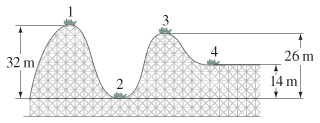# Problem: A roller-coaster car shown in the figure is pulled up to point 1 where it is released from rest. (a) Assuming no friction, calculate the speed at point 2.(b) Assuming no friction, calculate the speed at point 3.(c) Assuming no friction, calculate the speed at point 4.

###### FREE Expert Solution

In this problem, we are given the heights (in the image) and asked to calculate the velocity. The height and velocity are indicators of the energy possed by an object. Thus, this is a conservation of energy problem.

Law of conservation of energy:

$\overline{){{\mathbf{K}}}_{{\mathbf{i}}}{\mathbf{+}}{{\mathbf{U}}}_{{\mathbf{i}}}{\mathbf{+}}{{\mathbf{W}}}_{{\mathbf{nc}}}{\mathbf{=}}{{\mathbf{K}}}_{{\mathbf{f}}}{\mathbf{+}}{{\mathbf{U}}}_{{\mathbf{f}}}}$, where Wnc is the work done by non-conservative forces such as friction.

80% (435 ratings)###### Problem Details

A roller-coaster car shown in the figure is pulled up to point 1 where it is released from rest.(a) Assuming no friction, calculate the speed at point 2.

(b) Assuming no friction, calculate the speed at point 3.

(c) Assuming no friction, calculate the speed at point 4.

Frequently Asked Questions

What scientific concept do you need to know in order to solve this problem?

Our tutors have indicated that to solve this problem you will need to apply the Motion Along Curved Paths concept. You can view video lessons to learn Motion Along Curved Paths. Or if you need more Motion Along Curved Paths practice, you can also practice Motion Along Curved Paths practice problems.

What professor is this problem relevant for?

Based on our data, we think this problem is relevant for Professor Kolodiy's class at Kean University.